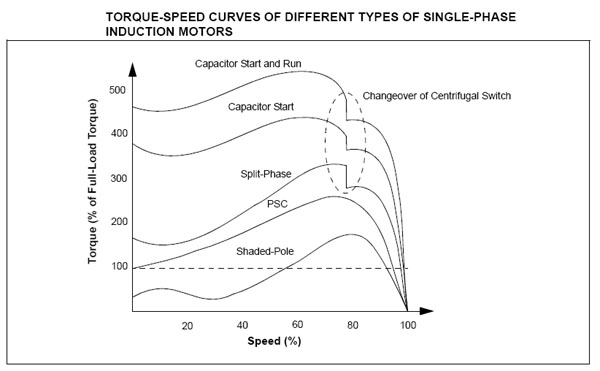## Torque-speed Characteristics Assignment Help

Assignment Help: >> Single Phase Induction Motors - Torque-speed Characteristics

Torque-speed Characteristics:

As seen from the axis of the winding, the MMF at any angle, θ, is

F = FP  cos θ

Where FP = Peak MMF, and

θ = Angle measured from winding axis.

We know that

FP  = FM  cos ωt

Where            FM = Maximum value of MMF. From Eqs. (60) and (61), we have

F =( ½) F M cos (θ - ωt ) + (½) FM cos (θ + ωt )

where  ½  FM cos (θ- ωt ) is the forward rotating field, F fd , and

½  FM   cos (θ+ ωt ) is the backward rotating field, Fbd

If the rotor rotates at the speed Nr, in the direction of Ffd, the slip of rotor with respect to the Ffd is then

S fd   = (Ns  - N)/ Ns  = S

Whereas the rotor slip with respect to the Fbd is

Sbd  = Ns  - (- Nr )/ Ns  = (2 - S )

Therefore the rotor slips with respect to the backward and forward rotating fields are different.

The torque-speed characteristics of a single-phase induction motor is illustrated in FigureFigure: Torque-speed Characteristics of a Single Winding Single-phase Induction Motor

When Nr = 0; S = 1, the two rotating fields have the same strength and generates equal and opposite torques resulting in net starting torque of zero value. However, If the rotor is made to run at speed Nr in the direction of the forward field, the two slips are how S and (2 - S). The result of weakening of one field and simultaneously strengthening of the other leads to a torque-speed characteristics like that of a three-phase induction motor in the speed region close to Ns.

Single-phase induction motors may be classified in the following way

1. Resistance split-phase motor

2. Capacitor split-phase motor

3. Capacitor-start motor

4. Two value capacitor motor.